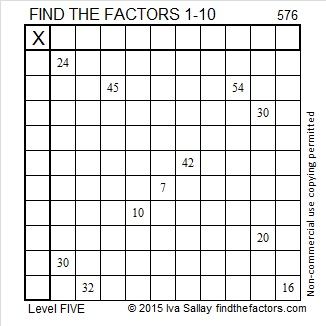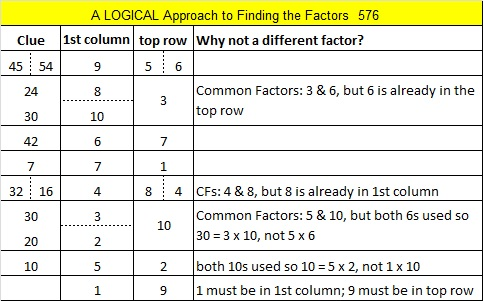# 576 and Level 5

The number formed from the last 2 digits of 576 is divisible by 4. That means 576 is also divisible by 4.

576 is made from 3 consecutive numbers so it is divisible by 3. Since the middle number, 6, is also divisible by 3, we know that 576 is also divisible by 9.

Either of the previous statements is enough to indicate that √576 can be reduced. In fact, boy, can it ever be reduced!

576 is the smallest number to have exactly 21 factors. (Only perfect squares have an odd number of factors.)

576 is also the sum of consecutive primes in two different ways:

• 283 + 293 = 576
• 137 + 139 + 149 + 151 = 576Print the puzzles or type the solution on this excel file: 10 Factors 2015-08-03

—————————————————————————————————

• 576 is a composite number.
• Prime factorization: 576 = 2 x 2 x 2 x 2 x 2 x 2 x 3 x 3, which can be written 576 = (2^6) x (3^2)
• The exponents in the prime factorization are 6 and 2. Adding one to each and multiplying we get (6 + 1)(2 + 1) = 7 x 3 = 21. Therefore 576 has exactly 21 factors.
• Factors of 576: 1, 2, 3, 4, 6, 8, 9, 12, 16, 18, 24, 32, 36, 48, 64, 72, 96, 144, 192, 288, 576
• Factor pairs: 576 = 1 x 576, 2 x 288, 3 x 192, 4 x 144, 6 x 96, 8 x 72, 9 x 64, 12 x 48, 16 x 36, 18 x 32 or 24 x 24
• 576 is a perfect square. √576 = 24—————————————————————————————————This site uses Akismet to reduce spam. Learn how your comment data is processed.